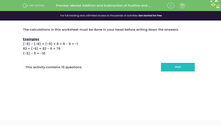# Add and Subtract Positive and Negative Numbers Mentally

In this worksheet, students will add and subtract positive and negative numbers in their heads.Key stage:  KS 3

Curriculum topic:   Number

Curriculum subtopic:   Use Four Operations for All Numbers

Difficulty level:#### Worksheet Overview

It might seem tough, but it's really good practice for solving problems quickly!

It is really important to learn the rule for negative numbers, so let's recap it here.

When two signs are written next to each other:

If the two signs are different, they make a negative sign.

If the two signs are the same, they make a positive sign.

This means that:

+ and - make a negative

- and + make a negative

+ and + make a positive

- and - make a positive

For example, when adding a negative number, you have + and - together, so the answer will be negative:

7 + -9 = 7 - 9 = -2

When subtracting a negative number, you have - and - together, so the answer will be positive:

3 - -5 = 3 + 5 = 8

So, let's have a look at some tough ones!

Examples

(-9) - (-8) = (-9) + 8 = 8 - 9 = -1

82 + (-6) = 82 - 6 = 76

(-5) - 5 = -10Has your brain given up? Don't panic, we'll go through them together.

Let's get started!!

### What is EdPlace?

We're your National Curriculum aligned online education content provider helping each child succeed in English, maths and science from year 1 to GCSE. With an EdPlace account you’ll be able to track and measure progress, helping each child achieve their best. We build confidence and attainment by personalising each child’s learning at a level that suits them.

Get started